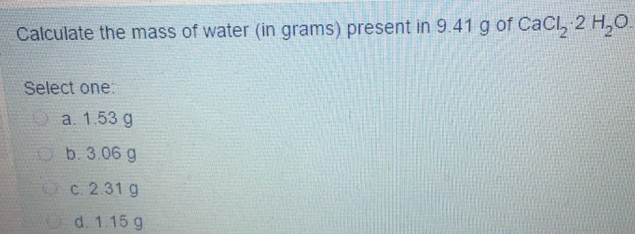# Problem: Calculate the mass of water (in grams) present in 9.41 g of CaCl_2 2 H_2O. Select one. a. 1.53 g b. 3.06 g c. 2.31 g d. 1.15 g

###### FREE Expert Solution
81% (421 ratings)###### Problem Details

Calculate the mass of water (in grams) present in 9.41 g of CaCl_2 2 H_2O. Select one.

a. 1.53 g b. 3.06 g c. 2.31 g d. 1.15 g